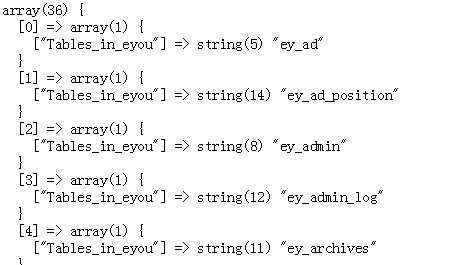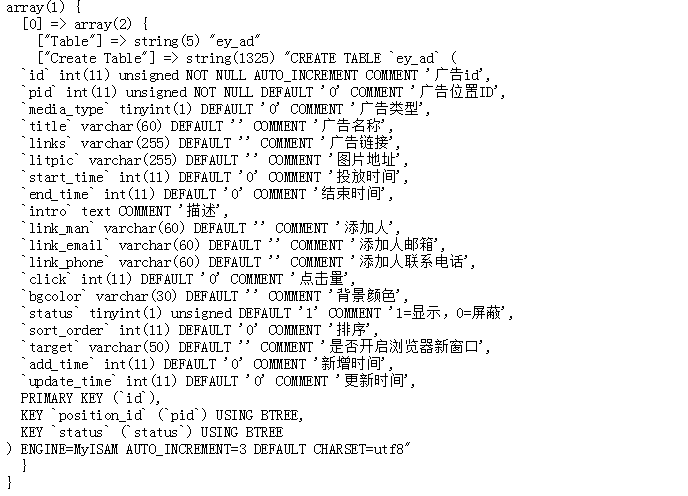• 备份程序一共只有两个类：数据库备份器(DbBak)和表备份器(TableBak)： 这个程序仅仅备份和恢复数据，使用方法很简单，实例化DbBak，然后调用bakupDb和restoreDb方法：
• 这是一个非常简单的利用php备份mysql数据库的类文件,我们只要简单的在dbmange中配置好连接地址用户名与数据库即可。 功能：实现mysql数据库分卷备份,选择表进行备份,实现单个sql文件及分卷sql导入 。
• 分享一个php数据库备份还原类。设置开启时间，启动PDO连接，储存表定义语句的数组，确定要备份的表，获取表单数据，写入文件。解析SQL文件为SQL语句数组
• 想在PHP后台管理直接能够备份数据库，于是想呀想，一直没有什么思路，一开始是考虑用php来访问服务器安装mysql的目录，比如 /usr/local/mysql/data目录，直接把下面对应的文件进行备份
• 主要介绍了php实现MySQL数据库备份与还原类,可实现针对MySQL数据库的导入与导出功能,并且包含了针对数据库操作时的加锁与解锁等功能,非常具有实用价值,需要的朋友可以参考下
• 基于thinkphp的mysql数据库备份与还原类，想在你thinkphp开发的项目中加入mysql数据库备份与还原功能的话，这是一个不错的选择
• 一个非常简单的利用php来备份mysql数据库的类文件,我们只要简单的在dbmange中配置好连接地址用户名与... 数据库备份（导出） 2. 数据库恢复（导入） 分别是主机，用户名，密码，数据库名，数据库编码
• 数据库中拿到要备份的数据表 //拿数据表 $sql = 'show tables';$tables_in_db = Db::query($sql); dump($tables_in_db);//打印 下面是打印结果 //遍历这个数组 调整一下结构 最后得到一个包含表名称的索引数组 ...
大致步骤如下:
从数据库中拿到要备份的数据表 //拿数据表
$sql = 'show tables';$tables_in_db = Db::query($sql);//你需要一个模型 (没有?下面附一个 这里是Tp5的模型) dump($tables_in_db);//打印
 下面是打印结果//遍历这个数组 调整一下结构  最后得到一个包含表名称的索引数组
$tables = array(); foreach ($tables_in_db as $k =>$v )
{
$tables[$k] = $v['Tables_in_'.$dbname];//$dbname 是xxx 则键名是 Tables_in_xxx }  拿到表结构 $sql = "show create table tableName";//tableName 要查看的表名
$create_table = Db::query($sql);
dump($create_table );  打印结果如下很关键, “Create Table” 字段里的值, 就是你的表结构!! 拿对应表的数据 (这个没什么可说的) 接下来是重点了 将表结构和对应数据记录保存到 一个名为 .sql 的文件里 还原的话就是从这个文件中拿sql语句 执行  下面是自己写的一个 一键备份, 和一键还原(很弱!=.= ,没有分包什么的) .sql 里的文件格式是模仿 Navicat for MySQL 导出的sql 文件 来写的 顺便附一个数据库操作类 https://blog.csdn.net/csdn_zhongwu/article/details/84257921 <?php /** * 数据库备份 */ class Backup { private$tables = array();//要备份的数据表
private $path;//文件保存路径 private$dbname;//database name;
private $model;//数据库模型对象 function __construct() {$this->model = new Db();//你需要一个模型  请自行解决
$this->path = ROOT.'/sql/';//常量ROOT 定义的项目根目录 请自行解决$this->dbname = DB_DBNAME;// database 名称 请自行解决
$this->check_path();$this->get_tables();
}

//备份方法
public function backupAll ()
{
if( $this->tables ) {$data = $this->genTitle(); foreach ($this ->tables as $table ) { //拿相关 create table 数据$ctable = $this->get_create_table($table);
//生成表结构
$data .=$this->get_table_structure($ctable); //表记录$data .= $this->get_table_records($table);
}
$filename =$this->path . time() .'.sql';
return file_put_contents($filename,$data);
}
}

//还原方法  拆分sql语句,  因为之前保存到文件中的语句都是以 ;\r\n 结尾的, 所以...
public function restore ($file) {$filename = $file; if( !file_exists($filename) )
{
return false;
}
$str = fread($hd = fopen($filename, "rb") , filesize($filename) );
$sqls = explode(";\r\n",$str);//所以... 这里拆分sql
if($sqls) { foreach($sqls as $sql) {$this->model ->query($sql);//逐条执行 } } fclose($hd);
return true;
}

//备份文件相关
public function getFileInfo()
{
$temp = array(); if( is_dir($this->path) )
{
$handler = opendir($this->path);
$num = 0; while ($file = readdir($handler) ){ if($file !== '.' && $file !== '..' ) {$filename = $this->path.$file;
$temp[$num]['name'] = $file;$temp[$num]['size'] = ceil(filesize($filename)/1024);
$temp[$num]['time'] = date("Y-m-d H:i:s" ,filemtime($filename));$temp[$num]['path'] =$filename;
$num ++; } } } return$temp;
}

//删除文件
public function delFile ($file) { if( file_exists($file) )
{
return unlink($file); } return false; } //sql 文件开头部分 可以省略 但 SET FOREIGN_KEY_CHECKS=0 最好有 private function genTitle() {$time = date("Y-m-d H:i:s" ,time());
$str = "/*************************\r\n";$str.= " * {$time} \r\n";$str.= " ************************/\r\n";
$str.= "SET FOREIGN_KEY_CHECKS=0;\r\n"; return$str;
}

private function get_tables ()
{
$sql = 'show tables'; if($data = $this->model ->fetchAll($sql) ) {
foreach ( $data as$val ) {
$this->tables[] =$val['Tables_in_'.$this->dbname]; } } } //返回一个数组, 0=>表名称 ,1=>表结构(Create Table) private function get_create_table ($table)
{
$sql = "show create table$table";
$arr =$this->model ->fetchOne($sql); return array_values($arr);
}

//生成表结构
private function get_table_structure ($ctable) {$str  = "-- ----------------------------\r\n";
$str .= "-- Table structure for {$ctable}\r\n";
$str .= "-- ----------------------------\r\n";$str .= "DROP TABLE IF EXISTS {$ctable};\r\n".$ctable.";\r\n\r\n";
return $str; } //表记录的sql语句拼接 当还原的时候 就是逐条插入记录 到对应的表 private function get_table_records ($table)
{
$sql = "select * from {$table}";
if( $data =$this->model ->fetchAll($sql) ) {$str = "-- ----------------------------\r\n";
$str.= "-- Records of$table \r\n";
$str.= "-- ----------------------------\r\n"; foreach ($data as $val ) { if($val ) {
//$keyArr = array();$valArr = array();
//这里看情况了,
foreach ( $val as$k => $v ) { //$keyArr[] = "".$k.""; //对单引号和换行符进行一下转义$valArr[] = "'".str_replace( array("'","\r\n"), array("\'","\\r\\n"), $v )."'"; } //$keys = implode(', ', $keyArr);$values = implode(', ', $valArr);$str .= "INSERT INTO {$table} VALUES ($values);\r\n";//省略了字段名称
}
}
$str .= "\r\n"; return$str;
}
return '';
}

private function check_path ()
{
if( !is_dir($this->path) ) { mkdir($this->path ,0777 ,true);
}
}
}


展开全文• 数据库备份和还原功能 安装 安装此扩展的首选方法是通过 。 无论运行 php composer.phar require --prefer-dist spanjeta/yii2-backup "*" 或添加 "spanjeta/yii2-backup": "*" 到composer.json文件的require部分...
•  // 数据库备份文件夹  var $record; // 换行符 private$ds = "n";  // 存储SQL的变量  public $sqlContent = ""; // 每条sql语句的结尾符 public$sqlEnd = ";";  /**  * ...
• php/** * 数据库备份还原类 * @author xialeistudio * Class DatabaseTool */class DatabaseTool{ private $handler; private$config = array( ‘host’ => ‘localhost’, ‘port’ => 3306, ‘user’ =>...
• 2、通过程序自带的数据库备份还原功能来操作，一些常见的PHP程序如DZ论坛等，后台都有数据库还原和备份的功能，方便我们转移空间数据。 3、如果您的数据库在朝暮数据购买，我们的管理面板支持一键备份和还原。点击...
• liunx服务器中数据库备份，今天要分享的是使用PHP定时每日备份数据库，保存sql文件，PHP脚本数据库备份
• <?php class Backup {  /**  * @var stores the options ... * @var stores the table structure inserts for every ... }这是一个很好用的php备份整个MySQL数据库类，需要的朋友可以下载使用
• phpweb如何备份恢复数据库？phpweb如何搬家？大家可以用自己收集的phpweb数据库备份搬家工具轻松搞定！
• 到此这篇关于php如何实现数据库的备份和恢复的文章就介绍到这了,更多相关php数据库备份和恢复内容请搜索软件开发网以前的文章或继续浏览下面的相关文章希望大家以后多多支持软件开发网！ 您可能感兴趣的文章:PHP...
• 本文实例讲述了TP5框架实现的数据库备份功能。分享给大家供大家参考，具体如下： 1、效果图 2、下载扩展类（ \extands\org\Baksql.php） 3、在 \public\static 里新建一个data 文件夹用来存放 .sql 的文件 4、...
• 文件：1、backup.php　数据库备份 　2、recovery.php　数据库恢复 　3、mydb.php　数据库类 功能：1、备份全部数据表； 　2、备份单个数据表； 　3、分卷备份全部数据表； 　4、分卷备份单个数据表； 　5、...
• mysql数据库备份工具（单文件php版本），适合数据库管理没有现成工具的情况下快速备份您的数据库。
• 数据库备份CLI应用 PS：这项工作的灵感来自我周围的许多人，尤其是创建的 。 这个主意 我希望有一个小型的cli应用程序，可以在需要时进行简单的修改即可用来备份数据库，所以我尝试了bach / shell，但我不喜欢它。 ...
• 推荐没有虚拟主机的小巧的Mysql数据库备份脚本（PHP
• 备份是一个Laravel软件包，它允许以简单的方式创建和恢复数据库备份。 新版本4. *适用于laravel 8 快速安装 首先通过Composer安装此软件包。 您可以运行： composer require paulvl/backup 4.* 或编辑项目的...
•  我在项目中随着数据库数据变多，以前的MySQL数据库备份方法不行了，就写了简单的PHP执行MySQL数据库备份和还原，利用mysqldump，mysqldump.exe和mysql.exe文件，PHP包的mysql文件夹中自带有，直接调用就好。...
                PHP执行Mysql数据库的备份和还原（利用mysqldump）
我在项目中随着数据库数据变多，以前的MySQL数据库备份方法不行了，就写了简单的PHP执行MySQL数据库备份和还原，利用mysqldump，mysqldump.exe和mysql.exe文件，PHP包的mysql文件夹中自带有，直接调用就好。但是mysqldump适合100G以下的数据库备份。
mysqldump命令将数据库中的数据备份成一个文本文件。表的结构和表中的数据将存储在生成的文本文件中。mysqldump命令的工作原理很简单。它先查出需要备份的表的结构，再在文本文件中生成一个CREATE语句。然后，将表中的所有记录转换成一条INSERT语句。然后通过这些语句，就能够创建表并插入数据。
PHP代码：
//设置时区
date_default_timezone_set("Asia/Shanghai");
/*********************************备份数据库start*********数据库大小100G以下*******************/
$db_user="***";//数据库账号$db_pwd="****";//数据库密码
$db_name="second";//数据库名$filename=date("Y-m-d")."-".time();
$name="E:/dataBackup/second_bear".$filename.".sql";//数据库文件存储路径
$exec="E:/MySQL/bin/mysqldump -u".$db_user." -p".$db_pwd." ".$db_name." > ".$name;$result=exec($exec); //前面要设置mysql执行文件的路径。 /*********************************备份数据库end****************************/ /*********************************还原数据库start******数据库大小100G以下**********************/$db_user="***";//数据库账号
$db_pwd="****";//数据库密码$db_name="second";//数据库名
$filename=data("Y-m-d")."-".time();$name="E:/dataBackup/second_bear".$filename.".sql";//数据库文件存储路径$exec="E:/MySQL/bin/mysql -u".$db_user." -p".$db_pwd." ".$db_name." < ".$name;
$result=exec($exec);
//print_r(\$result);
/*********************************还原数据库end****************************/
代码已测试。可以运行；有疑问或建议可以交流
展开全文mysqldump...